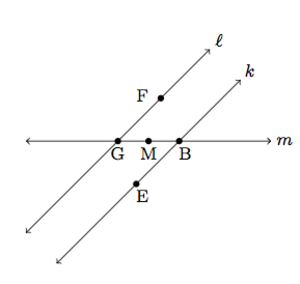# Congruent angles made by parallel lines and a transverse

Alignments to Content Standards: G-CO.C.9

In the picture below $\ell$ and $k$ are parallel:Show that the four angles marked in the picture are congruent.

## IM Commentary

The goal of this task is to prove congruence of vertical angles made by two intersecting lines and alternate interior angles made by two parallel lines cut by a transverse. Students will be familiar with these results from eighth grade geometry and here they will provide arguments with a level of rigor appropriate for high school. The solution (for alternate interior angles) uses properties of rigid motions and so it is assumed that students have already studied these. Two different arguments are provided, one using rotations and one using translations.

## Solution

We begin by showing that the pair of vertical angles where $k$ and $m$ meet are congruent. For this we mark several points on the diagram:We will show that $m(\angle DBC) = m(\angle EBA)$. Note that $\angle DBC$ and $\angle DBA$ are supplementary, together forming a straight angle.  So $m(\angle DBC) + m(\angle DBA) = 180$. Similarly, $\angle EBA$ and $\angle DBA$ together make line $k$ and they too are supplementary: $m(\angle EBA) + m(\angle DBA) = 180$. From these two equations we see that $m(\angle DBC) = m(\angle EBA)$. The other pair of vertical angles in the problem, made by $\ell$ and $m$, are congruent by the same reasoning.

To show that all four angles are congruent, it is now sufficient to show, in the picture below, that $m(\angle GBE) = m(\angle BGF)$.Here $B$ and $E$ are the same points as in the picure above and we have added points $F$ and $G$ as well as the midpoint $M$ of $\overline{BG}$. A 180 degree rotation of the plane about $M$ maps $B$ to $G$ and $G$ to $B$ since $M$ is the midpoint of $\overline{BG}$. If $t$ is the line parallel to $k$ and $\ell$ through $M$ then $t$ is mapped to itself by the 180 rotation with center $M$. The rotation maps parallel lines to parallel lines so this means that $k$ must map to the line through $G$ and parallel to $t$, that is $k$ maps to $\ell$. Similar reasoning shows that $\ell$ maps to $k$. This means that the 180 degree rotation with center $M$ interchanges $\angle GBE$ and $\angle BGF$, making these two angles congruent. Combining our congruent vertical angles and congruent alternate interior angles, we have shown that all four angles in the picture are congruent.

There is a second way to show that $m(\angle GBE) = m(\angle BGF)$ using translations and their properties. Suppose we apply a translation by $\overline{GB}$ to the plane. This translation maps $m$ to itself and maps $\ell$ to a line parallel to $\ell$ containing $B$. The only line parallel to $\ell$ through $B$ is $k$ so this means that $\ell$ maps to $k$. Translations preserve angles so this means that $m(\angle BGF) = m(\angle CBD)$ with notation as in the first picture. We already showed that $m(\angle CBD) = m(\angle GBE)$ and so we have shown that $m(\angle BGF) = m(\angle GBE)$.